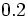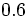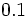# Limiting coefficient of static friction

## Definition

Given two surfaces, the limiting coefficient of static friction between them, denoted$\mu_s$ or$\mu_l$, is defined as the number such that the static friction between any two bodies with these two surfaces respectively as the surfaces of contact cannot exceed$\mu_sN$, where$N$ is the normal force between the two bodies.

The notion of limiting coefficient of static friction arises as part of the Coulomb model of friction.

## Units and dimensions

The limiting coefficient of static friction is a dimensionless scalar and does not need units.

## Range of values

The limiting coefficient of static friction typically takes values in the range of$0.2$ to$0.6$. In most cases, the limiting coefficient of static friction is less than$1$, but there could be situations where it is greater than$1$. For very slippery surfaces, the limiting coefficient of static friction may be less than$0.1$.

## Measurement

The limiting coefficient of static friction must be determined empirically, though measurement. Because a lot depends on the specific surfaces, the measurement may vary due to factors such as dampness, scratches, or minor changes to the surface.